Primitives

Overview

In Houdini, primitives refer to a unit of geometry, lower-level than an object but above points. Houdini supports several different types of primitives:

There is also a special primitive called a packed primitive that represents a lightweight reference to geometry stored somewhere else. This is very useful for copying/instancing and working with heavy geometry. Alembic files are represented in Houdini as packed primitives.

There are also several quadratic primitive types that represent geometric figures mathematically rather than as polygonal surfaces. These may be useful as lightweight geometry.

• Circle (defined by a single point, rotation, and radius).

• Sphere/ellipsoid (defined by a single point and radii).

• Tube/cone (defined by a single point, rotation, radii, and length).

Houdini’sCircle,Sphere, andTube have parameters to choose whether they create, for example, a "primitive sphere" or a polygonal sphere.

You can convert most geometry types between each other using theConvert SOP.

Polygons and meshes

Polygons are shapes constructed from a series of straight edges. These edges are defined by a series of vertices. Polygons are ideal for dealing with complex topologies that go beyond the four sided nature of meshes and NURBS surfaces.

A mesh is a collection of polygons with guaranteed ordering. It is much more efficient that the equivalent polygons, and unlike most regular polygons you can convert it directly to NURBS. You can also use theConvert surface node to convert mesh primitives to regular polygons when you want a nice layout of polygons in rows and columns.

Open/closed

A closed polygon shares its first and last vertex and is flagged internally as "closed". Only closed polygons are shaded.

Planar/non-planar

Planar polygons' vertices lie in the same plane in 3D space. Non-planar polygons have vertices that are not all on a single plane in 3D space.

Convex/concave

A polygon is convex if every infinite line in the same plane as it intersects it at most twice. Concave polygons have more complex shapes with "inset" points.

Use the tools on the Create shelf tab to create surfaces, and use the Primitive type pop-up menu on the operation controls toolbar to set the new surface to be created from polygons.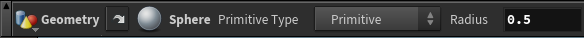Use the tools on the Polygon shelf tab to edit and reshape faces and polygonal surfaces.

Polygon soup

A polygon soup is a primitive that stores polygons using a compact memory representation and very efficiently stores faces whose primitive attributes are the same. Its in-memory representation allows almost all the memory of a large soup to be shared between nodes operating on the soup as long as the topology does not change (where regular polygons require each node to retain a copy of every polgyon). The sharing also makes memory caches more efficient, meaning many modeling and rendering operations will be faster as well as using less memory.

The more polygons in a soup, the greater the benefit over regular polygons. For simple geometry with few polygons, the management overhead of soups can make them slower than regular polygons, although soups will always use less memory.

You can convert geometry to a polygon soup using thePolysoup node.

Loading a soup from a file can be much faster than loading regular polygons (for Mantra as well as Houdini). You can load Alembic files as polygon soups. CreatingGrid,Sphere,Tube, andTorus shapes is faster when you use the option to create soups.

Along with the shape nodes, you can create soups withConvert,Convert Meta,Convert VDB,Convert Volume,Iso Offset, Iso Surface,Point Cloud Iso,Subdivide, andTetra Surface support creating polygon soups. You can use theSubdivide node to subdivide soups if you choose one of the OpenSubdiv methods.

Many (but not all) geometry nodes can operate "natively" on poly soups. You get the benefits of the soup’s sharing with operations that modify attributes, such asEdit,Transform,Sculpt,Attribute Transfer onto points, point Group,Attribute Create,Attribute Wrangle,Attribute Copy, computing point normals withFacet, pointUV Project,Paint,Partition, pointConnectivity, andRay, among others.

Some operations modifying vertex or primitive attributes may still work on polygon soups, but may have slightly different meaning than for polygons. For example, each soup is only one primitive, so the polygons in a soup can’t have their own primitive attributes. Also, polygon soups often do not have as many vertices as polygon primitives (they merge identical vertices). This means, for example,UV Project can’t compute vertex texture coordinates correctly, and cusping vertex normal isn’t supported.

Most operations that change polygon topology, such asPoly Extrude andPoly Split, don’t support soups, but a few do. For example, the Convex polygons option on theDivide node will convex a polygon soup, and will avoid adding any additional vertices, allowing the memory for the vertex attributes to be shared.

For nodes that don’t support soups, you can useConvert to convert a soup to regular polygons, and then convert the polygons back to a soup usingPolysoup. However, the benefits of polygon soups will usually be overwhelmed by the conversion cost, so in most cases you should just use regular polygons. (If you're doing this type of conversion back and forth in an HDA, you should set the Unload flag on all nodes whose output is polygons, so the SOP cache won’t hold onto the polygons longer than necessary.)

Polygon soup vs. Packed primitives vs. regular polygons

 Editing Regular polygons are supported by all operations in Houdini. Polygon soups are supported by many but not all operations in Houdini. The geometry of packed primitives is not editable or deformable. However you can transform them and set attributes on the points that represent the packed geometry. Memory usage Packed primitives are only loaded into memory as needed. For packed primitives that reference a disk file, they are streamed directly to the graphics card for display and to the renderer for rendering. The ongoing memory usage of a packed primitive can often be zero. Polygon soups have a much more compact memory representation than regular polygons. Regular polygons use the most memory of the three. Tips For small pieces of geometry, use regular polygons to avoid the overhead of polygon soups. If you need to use any operations that change topology or are otherwise don’t support polygon soups, use regular polygons all the way through – the cost of converting usually overwhelms any benefit from using soup for only part of a node chain. If you have large geometry you need to edit or deform, use polygon soups. If you only need to display and transform the geometry, store it on disk and use packed primitives.

Interpreting memory usage

You can see how much memory a node is using by pressingon the node. A pop-up will appear showing various ways of measuring the memory usage of the node:

Memory

The total amount of memory referenced by a particular detail. This memory may be shared with another node (for example, if you're using a polygon soup), so this isn’t necessarily how much memory you’ll save if you delete this node. It’s more a measure of how much stuff is passing through the node.

New

The amount of memory referenced by this node that is not referenced by any of its inputs. This indicates how much memory is used by this node. This number is not reported for nodes without inputs (generators).

Unique

The memory that is only referenced by this node (that is, not referenced by its inputs or outputs). This indicates how much memory you would save if you turned on the Unload flag on this node.

This display has some limitations:

• The memory referenced by packed primitives is not yet counted for performance reasons.

• The SOP cache manager and theCache SOP do not yet track memory usage correctly.

• Pressingon a subnet (such as a digital asset) only shows the memory used by the subnet itself, not the memory usage of the network inside the subnet.

Metaballs

Metaballs create an implicit surface computed around a set of points. Metaballs are like spheres centered around points, but when two metaballs are placed near each other their surfaces merge smoothly.Metaballs may be useful for representing blobby or weird surfaces. You can also change how the surface is computed, which may be useful to achieve some effect:NURBS and Bezier splines

• NURBS is an acronym for Non-Uniform Rational B-Spline. NURBS are smooth curves and surfaces defined by control vertices (CVs). See the Wikipedia definition for more information. The advantage of NURBS is that moving a CV reshapes surrounding curve/surface while maintaining the continuity of the curve/surface. This allows you to "pull and tug" on the CVs of the NURBS curve or surface to generate a desired shape without causing kinks or discontinuities.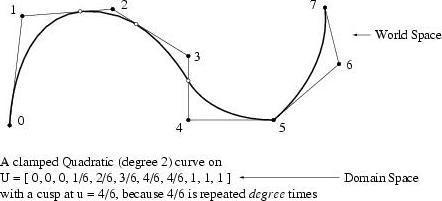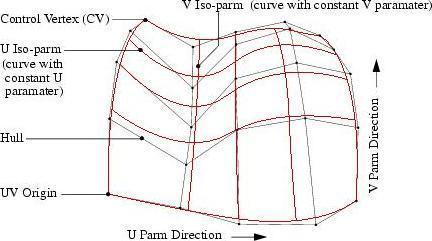• Bézier curves/surfaces are simpler than NURBS. See the Wikipedia definition for more information. Bézier curves allow discontinuities at the knots, making it easier to create corners but harder to create smooth continuous curves/surfaces.

Parameterization

The shape of a NURBS curve, given a set of CVs, is determined by the relative distance between knots. There are two types of knot parameterizations: uniform and chord length.

• In uniform parameterization, knots are spaced evenly.

• In chord length parameterization, the distances between knots are determined by the distances between successive CVs. Uniform parameterization is recommended for regular shapes while chord length is used for free-form shapes. A third type of parameterization, called "centripetal", is similar to chord length and is best suited for sharp curves.

Order/degree

Spline curves have an order , sometimes referred to instead as degree, which is one less than the order. The degree determines the potential smoothness of the line. A polyline has degree 1 (or "second order"). A curve with one control point between knots has degree 2 (AKA "third order" or "cubic"). The familiar Bézier curve, with two control points between knots, is degree 3 (AKA "fourth order" or "quadratic").

Houdini supports splines with degrees from 1 to 10. For animation and effects you’ll usually find that degree 2 (cubic) splines are sufficiently smooth and well behaved You will seldom need to use other degrees.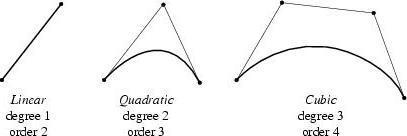Creating a sharp point in a NURBS curve

Sometimes you want to create a sharp corner in a NURBS curve. You can do this in three ways:

• Change the weight of a selected CV via the Curve > Parameters dialog in the Model Editor to something high like 10,000. This gives the CV so much "pull" that it draws the curve almost right through it.

• If you drag the two adjacent CVs of a cubic curve onto a middle CV, it will look like a sharp corner point. This is called raising the Multiplicity of the CV. Maximum CV multiplicity occurs when adjacent "degree" CVs overlap.

• Make "degree" knots identical. When this is done, it is called raising the multiplicity of the knot. You can do this in the Refine sop by choosing the Subdivision option, or in the Model Editor by selecting the Refine state and dividing with the middle mouse button ().

Houdini supports primitive spheres/ellipsoids, circles/ellipses, and tubes/cones. The shapes are defined by very simple mathematical parameters (for example, by height, and width for an ellipsoid) and a single center-point vertex. They can be moved, rotated, and scaled.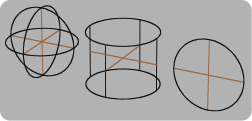Quadratic primitives are extremely lightweight, and so can be used for applications such as:

• Showing hundreds of thousands or millions of copies of simple shapes, as in instancing .

• Stand-in geometry.

• Envelope geometry (for example, for bouncing particles off of).

Many modeling tools will work on quadratic primitives, but the primitives do not have any topology so, for example, operators that act on surface points will not work with quadratic primitives.

Geometry

Understanding

• Describes how Houdini represents geometry using details, primitives, points, vertices, and attributes.

Modeling

• In Houdini you can not only edit the parameters of a surface node, you can reselect the geometry the operation applies to.

• Tools on the Polygon tab let you modify polygons in the scene view.

• Tools on the Model tab let you edit objects in the scene view.

Terrain

• How to use Houdini’s height field tools to generate realistic terrain.

• A heuristic workflow using the heightfield tools, based on experience generating realistic-looking terrain.

Fracturing

See RBD Fracturing in Dynamics.

Next steps

• The appearance editor mode of the data tree pane lets you edit various controls for object viewport/rendered appearance in one place.

Guru level

• You can mark a chain of compilable SOPs as a compiled block which can execute much faster in parallel.

• Many geometry (SOP) nodes allow you to use them in Python scripts to generate geometry programmatically.